• 现利用KMeans聚类实现LRFM模型来分析客户的价值，便于客户分群，针对性推广，提高销售额。LRFM模型定义：L:会员创建日期距离距离2014年7月25的时间间隔(单位：月 )R:会员最近一次购买时间距离2014年7月25的时间间隔...

1. 分析背景

这是一份某电商平台的销售数据，数据包含2010年4月22到2014年7月24的销售数据。分析该销售数据，可以发现客户价值。

现利用KMeans聚类实现LRFM模型来分析客户的价值，便于客户分群，针对性推广，提高销售额。

LRFM模型定义：L:会员创建日期距离距离2014年7月25的时间间隔(单位：月 )

R:会员最近一次购买时间距离2014年7月25的时间间隔(单位：月 )

F:会员购买次数

M:会员的总购买金额

2. 分析流程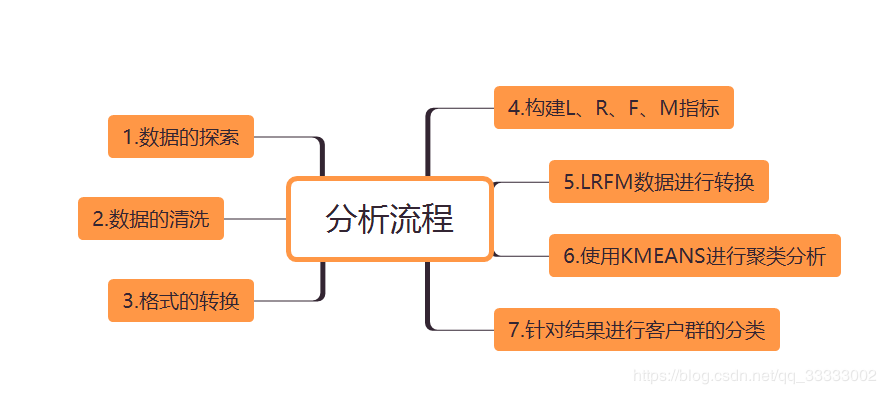3. 数据探索

3.1 导入相关包和读取数据import numpy as np

import pandas as pd

import matplotlib.pyplot as plt

from sklearn import preprocessing

from datetime import datetime

from sklearn.cluster import KMeans

plt.rcParams['font.sans-serif'] = 'SimHei'

%matplotlib inline

# 读取数据

engine='python')

# 查看行列

df.shape

输出：

3.2 查看表结构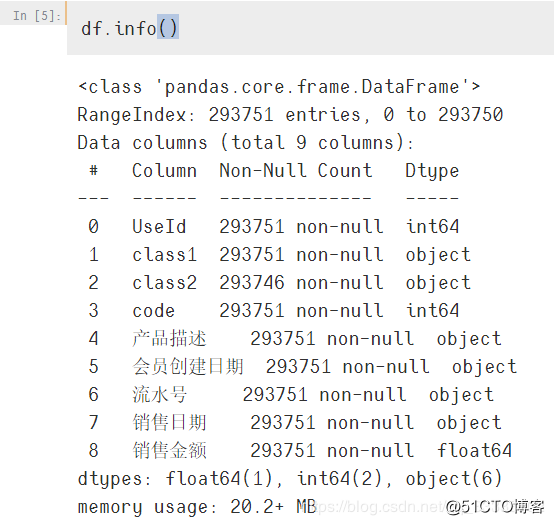从图可以看出这里的数据只有class2有缺失值，这里暂时不用提取这个指标，暂不清洗。

3.3 描述性分析查看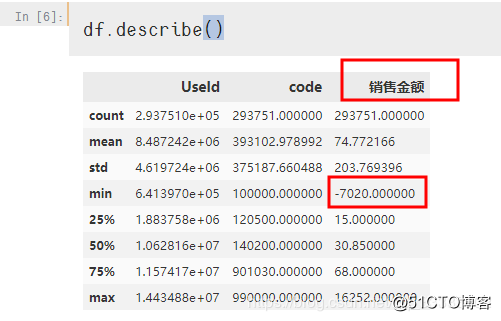这里销售金额为负数的情况，数据清洗的时候得把这些异常值过滤。

4. 数据清洗

4.1 将销售额<0的过滤掉# 销售金额有小于等于0的，直接过滤掉

# 这里有22542条数据

data = df[df['销售金额'] >0]

data.shape

输出：

4.2 会员创建日期、销售日期转换成datetime格式data['会员创建日期'] = pd.to_datetime(data['会员创建日期'])

data['销售日期'] = pd.to_datetime(data['销售日期'])

# 查看是否转换成功

data.info()

输出：

5. 构建L、R、F、M指标

5.1 提取有用指标L = 相对日期(这里我指定：2014年7月25) - 会员创建日期

R = 相对日期(这里我指定：2014年7月25) - 最晚(大)的销售日期

F = 用户购买的次数(这里针对流水号进行计数不同)

M = 用户购买的汇总金额

代买实现：# 计算L,再转换成月，这里转换成月，直接除于30天，保留两位小数

# L是最早的购买日期距离会员创建日期

data1 = data.groupby('UseId').agg({'会员创建日期': ['min'],

'销售日期': ['min', 'max'],

'销售金额':['sum'],

'流水号':['nunique']})

data1

输出：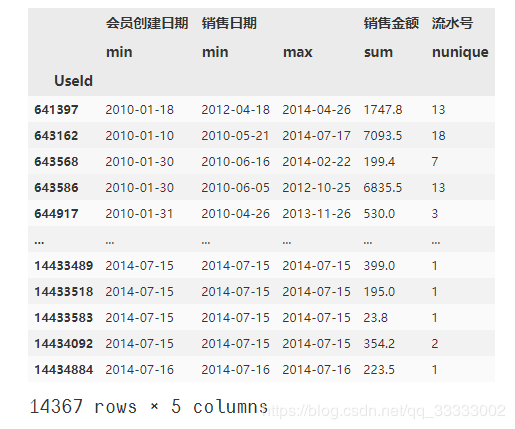删除一层列名，并重新进行命名：# 删除第一层的列名

data1.columns = [col for col in data1.columns]

# 重新命名列名

data1.columns = ['会员创建日期', '最早销售日期', '最晚销售日期', 'M', 'F']

data1

输出：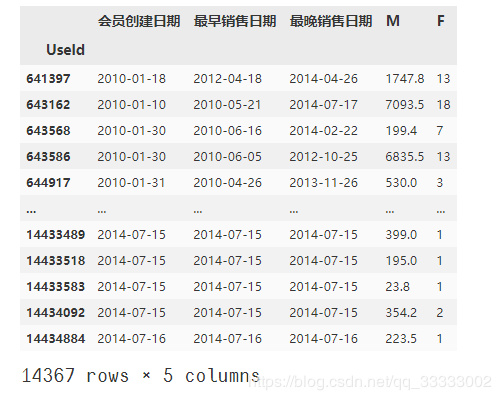M、F指标已经构建完成。

5.2 购买L、R指标# 先计算L,R,再转化成单位月

data1['L'] = datetime.strptime('2014-7-25', '%Y-%m-%d') - data1['会员创建日期']

data1['R'] = datetime.strptime('2014-7-25', '%Y-%m-%d') - data1['最晚销售日期']

# 将L、R转换成月做为单位

data1['L'] = data1['L'].apply(lambda x: round(x.days/30,3))

data1['R'] = data1['R'].apply(lambda x: round(x.days/30,3))

data1

输出结果：

提取有用的指标：LRFM_data = data1[['L', 'R', 'F', 'M']]

6. 进行L、R、F、M数据的Z-Score转换ss = preprocessing.StandardScaler()

ss_LRFM_data = ss.fit_transform(LRFM_data)

ss_LRFM_data

输出：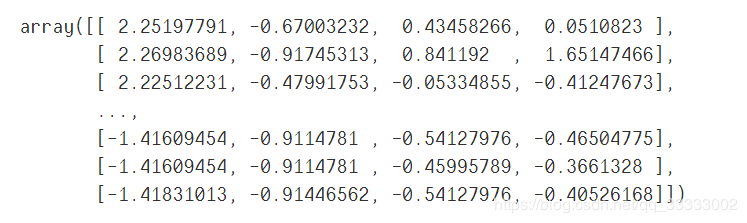7. 使用KMeans进行聚类分析# n_clusters聚类的个数

kmodel = KMeans(n_clusters=5, n_jobs=4)

kmodel.fit(ss_LRFM_data)

#查看聚类中心

kmodel.cluster_centers_

输出：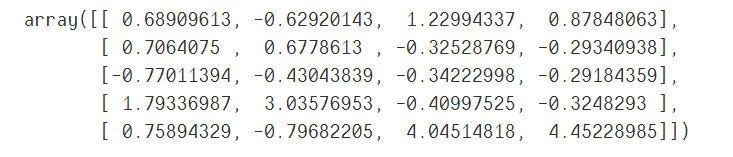将结果转成DataFrameclient_level = pd.DataFrame(kmodel.cluster_centers_,

index=['客户群1', '客户群2', '客户群3', '客户群4', '客户群5'],

columns=['L', 'R', 'F', 'M'])

client_level

输出：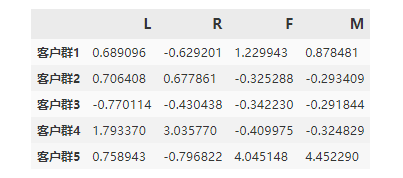8. 针对结果进行客户群的分类L越大，代表注册会员时间距离指定时间(2014年7月25)越长，代表老客户，该指标越大越好。

R越小，代表购买时间距离指定时间(2014年7月25)越短，R越小越好。

F越大，代表会员的购买次数越多。

M越大，代表会员购买的金额越多。

客户群1分析：

L大，R小，F大，M较大，这里判断是重要发展客户。

客户群2分析：

L大，R大，F小，M较小，这里判断是重要挽留客户。

客户群3分析：

L小，R小，F小，M小，这里判断是低价值客户。

客户群4分析：

L大，R大，F小，M小，这里判断是一般价值客户。

客户群5分析：

L大，R小，F大，M大，这里判断是重要保持客户。

展开全文• 数据分析实战——用RFM模型分析客户价值阿雷边学边教python数据分析第4期——数据可视化一、介绍什么是RFM模型和作用1.什么是RFM模型RFM模型是衡量客户价值的一种工具，该模型通过客户的RFM三项指标来描述该客户的...

数据分析实战——用RFM模型分析客户价值

阿雷边学边教python数据分析第4期——数据可视化

一、介绍什么是RFM模型和作用

1.什么是RFM模型

RFM模型是衡量客户价值的一种工具，该模型通过客户的RFM三项指标来描述该客户的价值状况，依据这三项指标划分8类客户。8种客户分类：2.RFM模型有什么用

通过RFM对客户价值分类，可以实现精准化营销，针对不同的客户群体，采取不同的策略

二、如何用python实现的思路和任务模块数据源需要4个字段：客户名称/ID，交易日期，成交量，成交金额

所以如果你有这样的数据，那么可以跟着来练习下RFM模型，或者用我提供的数据源来练习下。

实现思路和任务模块：

(1)计算出R

即已知交易日期，去计算出每个客户最近一次交易距离至今有多少天

(2)通过R给客户打分

根据客户最近一次交易距离至今的天数按照不同区间给出不同的分数

(3)计算出F

计算出每个客户的累计成交量

(4)通过F给客户打分

根据客户累计成交量按照不同区间给出不同的分数

(5)计算出M

计算出每个客户的累计成交额

(6)通过M给客户打分

根据客户累计成交额按照不同区间给出不同的分数

(7)计算RFM每一项的平均值

这么做是为了通过RFM每一项的平均值去判断客户在每一项上是高于平均值还是低于平均值，例如重要价值客户，就必须是RFM的三项都高于平均值才能被判断为重要价值客户。

(8)判断客户的每一项得分是否高于平均分

如果客户的某一项数值高于平均值，则返回1，否则返回0。也就是说1表示客户的某一项数值高于平均值，0表示客户的某一项数值低于平均值。1当做+，0当做-

(9)通过客户的比较结果对客户分类

比如三项都是111，则对于这个客户我们会判定为重要价值客户，依此类推。

(10)用图表呈现结果，并得出结论与建议

三、实战操作

在jupyter notebook上演示

展开全文• 本文由作者我偏笑发布于社区 三年前有幸接触俞老师的交易模型，是我得以构建起自己产品方法论的契机与起点。 随着阅读与实践积累，我对交易的理解逐步加深，一套更加完整的交易理论隐约浮现...

本文由作者 我偏笑 发布于社区
三年前有幸接触俞老师的交易模型，是我得以构建起自己产品方法论的契机与起点。

随着阅读与实践积累，我对交易的理解逐步加深，一套更加完整的交易理论隐约浮现在我脑海中，本文将尝试将其勾勒出来。
囿于实践，文中疏漏之处在所难免，能作抛砖引玉之用也好。
这一交易理论共分为微观、中观、宏观三个层次，分别称为行为模型、价值模型、市场模型：

• 行为模型：关注个体行为动机。

• 价值模型：关注交易如何创造价值及价值如何分配。

• 市场模型：关注如何设计市场使其创造更大价值。

01
行为模型

行为模型建立在这样一个前提假设上：人的任何有意识行为都是付出预期成本，购买预期收益。

行为模型的关键公式是：

某一个体做出某一有意识行为的动机强度

= 主观效用 - 直接成本 - 交易成本 - 机会成本

不严谨地说：

• 主观效用指，个体主观预期做出某一行为后的「满足感」。

• 直接成本指，交易中「付给对方的成本」。

• 交易成本指，交易中「付出但对方未得到的成本」。

• 机会成本指，本可得到，但放弃了的「最大代价」。

这里不仅效用为主观量，直接成本、交易成本、机会成本均为个体的主观判断。

举个例子，比如决策要不要跟朋友一起吃晚饭，做出这一行为的动机强度等于：

主观效用：预期跟朋友一起吃饭得到的快乐与满足感

减直接成本：吃饭所需要付出的金钱、时间、体力、脑力成本等

减交易成本：为了赶去目的地打车等所需要付出的金钱、时间、体力、脑力成本等

减机会成本：一个人在家吃饭所能得到的满足感，减去对应的直接成本&交易成本

行为模型具有两个关键作用，一个是预测他人的行为，另一个是理解自己的动机

在预测他人行为上，确实，由于这一公式中所有变量均为主观量，所以很难据此完全准确的预测一个自然人的行为，但它却可以有效预测群体（群体遵循大数定律）行为，或特殊个体（角色属性大于自然属性，如司机、骑手）的行为。

行为模型于我而言更大的作用，其实是帮助我更深入的理解自己。每当我产生某一动机或情绪的时候，我便会用其反向拆解情绪与动机的来源，这让我越来越清晰的意识到了自己在意的究竟是什么。

02
价值模型

价值模型是在行为模型解释个体行为的基础上，引入微观经济学，尝试进一步解释涉及多个体的交易行为如何创造价值、以及创造出的价值如何在各交易方间进行分配。

价值模型建立在「主观价值论」的前提上。

与客观价值论相对，主观价值论认为商品/服务并没有客观的内在价值，它的价值完全取决于人对它的主观判断。

如果价值是客观的，那交易便是一种零和博弈，若成交价高于价值，则供给方获益；若成交价低于价值，则需求方获益。这显然与常识不符，比如我去商店买了个苹果，是我亏了，还是商店亏了呢？

而如果价值是主观的，需求方与供给方对同一商品/服务将存在不同估值。需求方有自己的最高愿付价格，供给方有自己的最低愿售价格，当需求方的最高愿付价格高于供给方的最低愿售价格时，交易存在达成的可能性。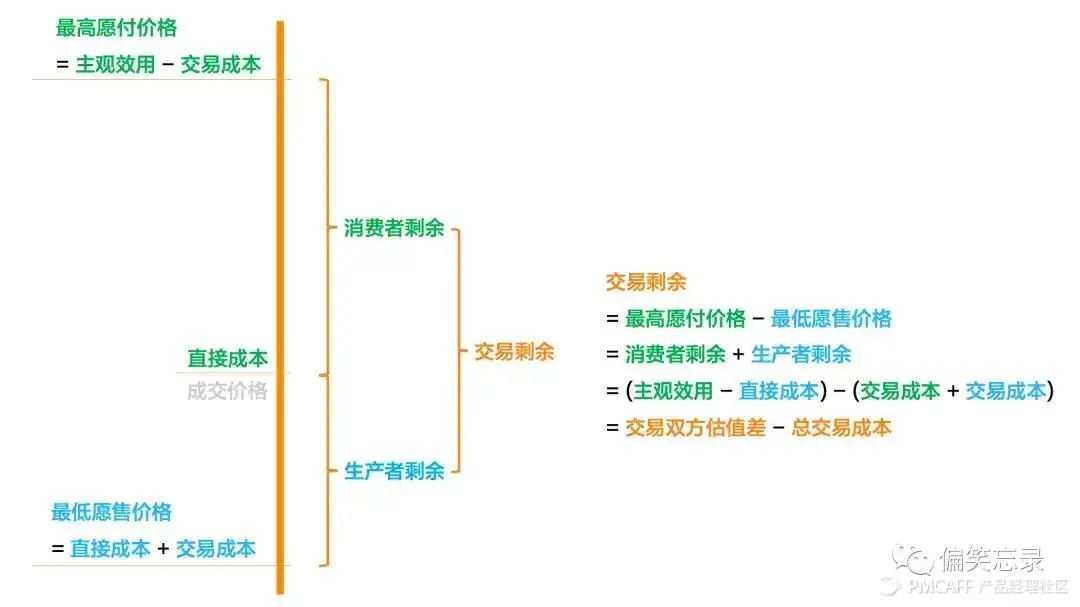如上图，结合行为模型，需求方的最高愿付价格受主观效用与（需求方的）交易成本影响，供给方的最低愿售价格受直接成本与（供给方的）交易成本影响。另外，这里的愿付价格与愿售价格根据交易性质的不同，未必均会用金钱来衡量（如时间、注意力等均可被交易）。

最高愿付价格与成交价格（即需求方的直接成本）间的差，被称为消费者剩余，即需求方在这次交易中得到的价值；成交价格与最低愿售价格间的差，被称为生产者剩余，即供给方在这次交易中得到的价值。

消费者剩余与生产者剩余相加，等于这次交易产生的交易剩余，也即这次交易新创造出的总价值。

在这类传统交易场景中，交易创造的价值等于供需双方对于同一商品/服务的估值差，减去双方的总交易成本。

如果我们进一步抽象，纯粹以价值视角看，任何一次涉及多方的交易都可以被拆分成两个阶段：价值创造价值分配

在价值创造阶段，第一个关键公式为：

交易为各个角色直接创造的价值

= 新主观效用 - 旧主观效用 - 交易成本

这一公式与俞老师的用户价值公式（用户价值 = 新体验 - 旧体验 - 替换成本）等价。

第二个关键公式为：

一次交易创造出的总价值

= Σ交易为各个角色直接创造的价值

自然地，交易创造出的总价值等于这次交易为各个角色创造出的价值之和。

而价值分配阶段的意义，在于将本次交易创造出的总价值，有效率地分配给参与交易的各个角色。

价值模型引导我们不再狭隘的考虑每一方是否得益，而是高屋建瓴的去思考交易的两个关键问题：

• 交易是否创造了新价值？

• 交易创造出的价值如何分配？

03
市场模型

市场模型是在行为模型与价值模型的基础上，思考如何依照交易原理，洞察、理解、设计市场，使其可持续、且尽可能多地创造价值

市场模型建立在对多边交易市场的解构上，理解一个多边交易市场通常可以从全局与局部两个视角入手。

全局视角需要关注两点：

第一，主导市场的商业平台为了自身存续，需要关注「财务状况」，如营收、毛利和成本构成等；

第二，因市场「多边」性质的存在，平台需确保市场「生态平衡」，如各边的相对规模是否合理等。

局部视角则聚焦于：

参与市场交易的各边（如网约车市场有司机、乘客两边），关注其「体验」「效率」「增长」「规模」。

体验关注某一边「能从市场中得到什么」，包含正向体验建设与负向体验治理；而效率则关注他们「能为市场提供什么」；增长与体验共同决定了规模。

基于价值模型，为实现价值创造的最大化，需要尽可能让更多交易发生，且最大化每次交易产生的交易剩余。由此，针对具有上述结构的多边交易市场，平台的关键任务有两个：

• 扩大有效规模

• 提高交易效率

扩大有效规模指，各边规模协调增长，且质量提升。

之所以强调「有效」，是由于不协调（如供给/需求过剩下，仍持续扩大供给/需求规模），低质量（如过高愿售价格的供给方，或过低愿付价格的需求方）的规模增长并不能带来更多交易。前文结构中的各边体验、增长，是决定市场能否扩大有效规模的关键。

提高交易效率指，最大化单位市场规模下创造的价值量（基本等同于最大化每次交易产生的交易剩余），与前文结构中的各边效率强相关。

依照价值模型，针对市场中任意一对供需双方，提高交易效率关键抓手有三类：

• 提高需求方最高愿付价格

• 降低供给方最低愿售价格

• 优化供需匹配

由于需求方的最高愿付价格 = 主观效用 - 交易成本，所以提升最高愿付价格也需要从提升主观效用与降低交易成本入手。

如国内网约车市场，通过排队给予了乘客应答确定性，提升了主观效用，进而提高了乘客的最高愿付价格（表现为乘客愿等时长提升）。

同理，降低供给方的最低愿售价格也要从降低直接成本与降低交易成本入手。

同样以网约车市场为例，若通过有效的调度算法，将司机提前调往热区，便能降低司机接单所需要付出的交易成本（缩短了接驾距离），进而降低其最低愿售价格（表现为接单率提升，相同价格的订单，过去不接，但现在接了）。

优化供需匹配指，由于信息不完全及其他约束条件的限制，平台在撮合交易时通常会存在以下两类问题：

1）错估需求方的最高愿付价格与供给方的最低愿售价格，产生错配，进而导致交易失败。

如将一个司机以5分钟接驾时长匹配给了一个愿等3分钟的乘客，而非以7分钟接驾时长匹配给另一个愿等10分钟的乘客。

2）任何交易的达成，都以供需双方本可达成的其它交易为代价。不当匹配会缩小交易双方的平均估值差，且带来更高交易成本。

如过早为乘客指派了远接驾司机，但随后，司机附近出现了更近的新乘客，而乘客附近也出现了更近的新司机。

供需匹配的优化可以从全局出发，尽可能减少错配，促成更多交易，且优化匹配质量，提升交易效率。

总体而言，市场模型以行为模型与价值模型为基础，是这一交易理论的宏观部分，也是更贴近真实实践场景的部分，它尝试为所有多边交易市场的分析与设计寻找出一套具有共性的方法，本文仅一己之见，希望对你有帮助。

↘好文推荐：
对账系统设计详解（上）

大厂没有方法论（上）
阿里设计师出品！B端产品文案指南

点个“在看”吧

展开全文• 客户生命周期可分为四个...在营销活动之前，需要对客户的潜在价值进行预测，或分析不同客户特征对客户价值的影响程度，这就涉及数值预测的相关方法，比较常用的就是线性回归模型 1. 线性回归 1.1 简单线性回归 使用st

客户生命周期可分为四个阶段：潜在客户阶段响应客户阶段既得客户阶段流失客户阶段

本章整体是一个客户价值预测的案例，背景是某信用卡公司在地推活动之后，获取了大量客户的信用卡申请信息，其中一个部分客户顺利开卡，并且有月消费记录，而另外一部分客户没有激活信用卡。公司的营销部门希望对潜在消费能力高的客户进行激活卡普的影响活动。在营销活动之前，需要对客户的潜在价值进行预测，或分析不同客户特征对客户价值的影响程度，这就涉及数值预测的相关方法，比较常用的就是线性回归模型

1. 线性回归

1.1 简单线性回归

使用statsmodel实现简单线性回归

本案例使用汽车贷款数据，相应字段如下

字段名中文含义
idid
Acc是否开卡(1=已开通)
avg_exp月均信用卡支出（元）
avg_exp_ln月均信用卡支出的自然对数
gender性别(男=1)
Age年龄
Income年收入（万元）
Ownrent是否自有住房（有=1；无=0)
Selfempl是否自谋职业(1=yes, 0=no)
dist_home_val所住小区房屋均价(万元)
dist_avg_income当地人均收入
high_avg高出当地平均收入
edu_class教育等级：小学及以下开通=0，中学=1，本科=2，研究生=3

本案例需要对用户月均信用卡支出建立预测模型，考虑到该变量与收入可能存在线性关系，因此可以先使用收入Income作为解释变量，建立简单线性回归模型

引入相应的包

%matplotlib inline

import numpy as np
import pandas as pd
import matplotlib.pyplot as plt
import seaborn as sns
import statsmodels.api as sm
from statsmodels.formula.api import ols
from pandas.core import datetools

#用于设置pandas数据框最大的显示列数，超过该显示列数会使用省略号来代替
pd.set_option('display.max_columns', None)

读取数据

# skipinitialspace，忽略分隔符后的空白（默认为False，即不忽略）

输出结果通过简单的观察和分析，可以得到以下数据清洗策略：

1）月均信用卡支出avg_exp为空值的是没有开卡（Acc = 0）的客户，因此使用avg_exp非空的数据进行建模，使用模型预测那些avg_exp为空的数据

2）Acc是用于代表用户时候开卡，只有开卡用户才会有信用卡支出，因此Acc不能进入模型

3）age2Age的平方，在这个案例意义不大，因此可以删除

4）avg_exp_In是月均信用卡支出avg_exp的对数值

5）字段字段id显然不能进入模型

数据清洗和描述性统计分析

exp = raw[raw['avg_exp'].notnull()].copy().iloc[:,2:].drop('age2',axis=1)

exp_new = raw[raw['avg_exp'].isnull()].copy().iloc[:,2:].drop('age2',axis=1)

# 进行变量的描述性统计分析
exp.describe(include='all')

相关性分析
要进行线性回归分析，首先要确认解释变量和被解释变量之间存在线性关系（或进过转换后存在线性关系）

#散点图
exp.plot('Income', 'avg_exp', kind='scatter')
plt.show()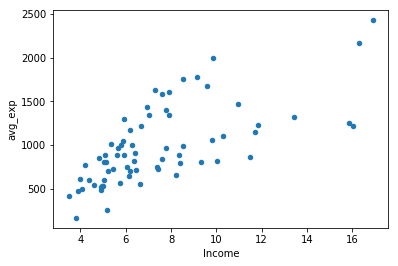exp[['Income', 'avg_exp', 'Age', 'dist_home_val']].corr(method='pearson')相关性分析可以判断出avg_expIncome确实存在较高的相关性，可以用简单线性回归来建立模型

lm_s = ols('avg_exp ~ Income', data=exp).fit()
# 输出一元线性回归系数
print(lm_s.params)

本段使用了statsmodel中的ols函数，该函数需要传入一个字符串作为参数（也被称为formula公式），该字符串使用波浪线“~”来分隔被解释变量和解释变量

ols函数返回一个模型（最小二乘法），该模型使用fit方法进行训练。训练完成的模型使用params属性保存解释变量的系数与方程的截距

In:

lm_s = ols(‘avg_exp ~ Income+Age+dist_home_val’, data=exp).fit()
lm_s.summary()

In:

lm_s.summary()

In:

pd.DataFrame([lm_s.predict(exp), lm_s.resid], index=[‘predict’, ‘resid’]

In:

lm_s.predict(exp_new)[:5]

In:

lm_m = ols(‘avg_exp ~ Age + Income + dist_home_val + dist_avg_income’,
data=exp).fit()
lm_m.summary()

In:

‘’‘forward select’’’
def forward_select(data, response):
remaining = set(data.columns)
remaining.remove(response)
selected = []
current_score, best_new_score = float(‘inf’), float(‘inf’)
while remaining:
aic_with_candidates=[]
for candidate in remaining:
formula = “{} ~ {}”.format(
response,’ + '.join(selected + [candidate]))
aic = ols(formula=formula, data=data).fit().aic
aic_with_candidates.append((aic, candidate))
aic_with_candidates.sort(reverse=True)
best_new_score, best_candidate=aic_with_candidates.pop()
if current_score > best_new_score:
remaining.remove(best_candidate)
selected.append(best_candidate)
current_score = best_new_score
print (‘aic is {},continuing!’.format(current_score))
else:
print (‘forward selection over!’)
break

formula = "{} ~ {} ".format(response,' + '.join(selected))
print('final formula is {}'.format(formula))
model = ols(formula=formula, data=data).fit()
return(model)

In:

data_for_select = exp[[‘avg_exp’, ‘Income’, ‘Age’, ‘dist_home_val’,
‘dist_avg_income’]]
lm_m = forward_select(data=data_for_select, response=‘avg_exp’)
print(lm_m.rsquared)

ana1 = lm_s

In:

exp[‘Pred’] = ana1.predict(exp)
exp[‘resid’] = ana1.resid
exp.plot(‘Pred’, ‘resid’,kind=‘scatter’)
plt.show()

遇到异方差情况,教科书上会介绍使用加权最小二乘法，但是实际上最常用的是对被解释变量取对数

ana1 = ols(‘avg_exp ~ Income’, exp).fit()
ana1.summary()

In:

ana2 = ols(‘avg_exp_ln ~ Income’, exp).fit()
exp[‘Pred’] = ana2.predict(exp)
exp[‘resid’] = ana2.resid
exp.plot(‘Income’, ‘resid’,kind=‘scatter’)
#ana2.summary()
#plt.show()

In:

exp[‘Income_ln’] = np.log(exp[‘Income’])

In:

ana3 = ols(‘avg_exp_ln ~ Income_ln’, exp).fit()
exp[‘Pred’] = ana3.predict(exp)
exp[‘resid’] = ana3.resid
exp.plot(‘Income_ln’, ‘resid’,kind=‘scatter’)
plt.show()
#%%
ana3.summary()

In:

r_sq = {‘exp~Income’:ana1.rsquared, ‘ln(exp)~Income’:ana2.rsquared,
‘ln(exp)~ln(Income)’:ana3.rsquared}
print(r_sq)

In:

exp[‘resid_t’] = (exp[‘resid’] - exp[‘resid’].mean()) / exp[‘resid’].std()

In:

exp[abs(exp[‘resid_t’]) > 2]

In:

exp2 = exp[abs(exp[‘resid_t’]) <= 2].copy()
ana4 = ols(‘avg_exp_ln ~ Income_ln’, exp2).fit()
exp2[‘Pred’] = ana4.predict(exp2)
exp2[‘resid’] = ana4.resid
exp2.plot(‘Income’, ‘resid’, kind=‘scatter’)
plt.show()

In:

ana4.rsquared

In:

from statsmodels.stats.outliers_influence import OLSInfluence

In:

exp2[‘dist_home_val_ln’] = np.log(exp2[‘dist_home_val’])
exp2[‘dist_avg_income_ln’] = np.log(exp2[‘dist_avg_income’])

ana5 = ols(’’‘avg_exp_ln ~ Age + Income_ln +
dist_home_val_ln + dist_avg_income_ln’’’, exp2).fit()
ana5.summary()

In:

ana5.bse # The standard errors of the parameter estimates

In:

def vif(df, col_i):
cols = list(df.columns)
cols.remove(col_i)
cols_noti = cols
formula = col_i + ‘~’ + ‘+’.join(cols_noti)
r2 = ols(formula, df).fit().rsquared
return 1. / (1. - r2)

In:

exog = exp2[[‘Income_ln’, ‘dist_home_val_ln’,
‘dist_avg_income_ln’]]

for i in exog.columns:
print(i, ‘\t’, vif(df=exog, col_i=i))

In:

exp2[‘high_avg_ratio’] = exp2[‘high_avg’] / exp2[‘dist_avg_income’]

In:

exog1 = exp2[[‘high_avg_ratio’, ‘dist_home_val_ln’,
‘dist_avg_income_ln’]]

for i in exog1.columns:
print(i, ‘\t’, vif(df=exog1, col_i=i))

In:

var_select = exp2[[‘avg_exp_ln’, ‘high_avg_ratio’,
‘dist_home_val_ln’, ‘dist_avg_income_ln’]]
ana7 = forward_select(data=var_select, response=‘avg_exp_ln’)
print(ana7.rsquared)

In:

formula8 = ‘’’
avg_exp_ln ~ dist_avg_income_ln + dist_home_val_ln +
C(gender) + C(Ownrent) + C(Selfempl) + C(edu_class)
‘’’
ana8 = ols(formula8, exp2).fit()
ana8.summary()

In:

formula9 = ‘’’
avg_exp_ln ~ dist_avg_income_ln + dist_home_val_ln +
C(Selfempl) + C(gender):C(edu_class)
‘’’
ana9 = ols(formula9, exp2).fit()
ana9.summary()

In:

lmr = ols(‘avg_exp ~ Income + dist_home_val + dist_avg_income’,
data=exp).fit_regularized(alpha=1, L1_wt=0)

lmr.summary()

In:

lmr1 = ols(‘avg_exp ~ Age + Income + dist_home_val + dist_avg_income’,
data=exp).fit_regularized(alpha=1, L1_wt=1)
lmr1.summary()

In:

from sklearn.preprocessing import StandardScaler

continuous_xcols = [‘Age’, ‘Income’, ‘dist_home_val’,
‘dist_avg_income’] # 抽取连续变量
scaler = StandardScaler() # 标准化
X = scaler.fit_transform(exp[continuous_xcols])
y = exp[‘avg_exp_ln’]

In:

from sklearn.linear_model import RidgeCV

alphas = np.logspace(-2, 3, 100, base=10)

Search the min MSE by CV

rcv = RidgeCV(alphas=alphas, store_cv_values=True)
rcv.fit(X, y)

In:

print(‘The best alpha is {}’.format(rcv.alpha_))
print(‘The r-square is {}’.format(rcv.score(X, y)))

In:

X_new = scaler.transform(exp_new[continuous_xcols])
np.exp(rcv.predict(X_new)[:5])

In:

cv_values = rcv.cv_values_
n_fold, n_alphas = cv_values.shape

cv_mean = cv_values.mean(axis=0)
cv_std = cv_values.std(axis=0)
ub = cv_mean + cv_std / np.sqrt(n_fold)
lb = cv_mean - cv_std / np.sqrt(n_fold)

plt.semilogx(alphas, cv_mean, label=‘mean_score’)
plt.fill_between(alphas, lb, ub, alpha=0.2)
plt.xlabel(" a l p h a \\alpha ")
plt.ylabel(“mean squared errors”)
plt.legend(loc=“best”)
plt.show()

rcv.coef_

In:

from sklearn.linear_model import Ridge

ridge = Ridge()

coefs = []
for alpha in alphas:
ridge.set_params(alpha=alpha)
ridge.fit(X, y)
coefs.append(ridge.coef_)

In:

ax = plt.gca()

ax.plot(alphas, coefs)
ax.set_xscale(‘log’)
plt.xlabel(‘alpha’)
plt.ylabel(‘weights’)
plt.title(‘Ridge coefficients as a function of the regularization’)
plt.axis(‘tight’)
plt.show()

In:

ridge.set_params(alpha=40)
ridge.fit(X, y)
ridge.coef_

In:

ridge.score(X, y)

In:

np.exp(ridge.predict(X_new)[:5])

In:

from sklearn.linear_model import LassoCV

lasso_alphas = np.logspace(-3, 0, 100, base=10)
lcv = LassoCV(alphas=lasso_alphas, cv=10) # Search the min MSE by CV
lcv.fit(X, y)

print(‘The best alpha is {}’.format(lcv.alpha_))
print(‘The r-square is {}’.format(lcv.score(X, y)))

In:

from sklearn.linear_model import Lasso

lasso = Lasso()
lasso_coefs = []
for alpha in lasso_alphas:
lasso.set_params(alpha=alpha)
lasso.fit(X, y)
lasso_coefs.append(lasso.coef_)

In:

ax = plt.gca()

ax.plot(lasso_alphas, lasso_coefs)
ax.set_xscale(‘log’)
plt.xlabel(‘alpha’)
plt.ylabel(‘weights’)
plt.title(‘Lasso coefficients as a function of the regularization’)
plt.axis(‘tight’)
plt.show()

lcv.coef_

In:

from sklearn.linear_model import ElasticNetCV

l1_ratio = [.1, .5, .7, .9, .95, .99]

encv = ElasticNetCV(l1_ratio=l1_ratio)
encv.fit(X, y)

In:

print(‘The best l1_ratio is {}’.format(encv.l1_ratio_))
print(‘The best alpha is {}’.format(encv.alpha_))
#%%

展开全文python 线性回归
• 在营销活动之前，需要对客户的潜在价值进行预测，或分析不同客户特征对客户价值的影响程度，这就涉及数值预测的相关方法，比较常用的就是线性回归模型 1. 线性回归 线性回归中，变量分为预测变量与python 线性回归
• 如何利用Python实现自动化股票价值检测，完成对市场的精确定位和战略选择python 爬虫 jupyter
• 一、数仓模型优化-如何判断一个数据模型的好坏 1.完善度 汇总数据能直接满足多少查询需求，即应用层访问汇总层数据的查询比例 跨层引用率：ODS层直接被DWS/ADS/DM层引用的表，占所有ODS层表比例 可以快速响应...
• 相比于千人千面的个性化运营，基于RFM用户价值分群实现简单、开发周期短，更能从整体层面判断用户的价值。 一.什么是RFM模型？ R代表消费时间间隔（Recency），指用户最近一次发生购买行为，距离今天的天数。F代表...
• 3月20日，北京智源人工智能研究院举办“智源悟道1.0 AI研究成果发布会暨大规模预训练模型交流论坛”。北京市科委、中关村管委会副主任许心超出席会议并致辞。北京大学、清华大学、中国人民大学...大数据 人工智能 机器学习 数据分析 自然语言处理
• 这个看似笑话的经历，背后隐藏着价值判断已经流于形式。 也许你会要求业务方直接给出需求优先级列表，把任务分为P0到P5，这样的划分除了按需求本质的优先级还同时考虑了开发资源、开发难度等问题。毫不夸张地说，我...产品经理 产品需求 产品路线图 优先级排序
• ARCH模型

2021-01-14 02:43:59
ARCH模型(Autoregressive conditional heteroskedasticity model)[编辑]什么ARCH模型?ARCH模型由美国加州大学圣迭哥分校罗伯特·恩格尔(Engle)教授1982年在《计量经济学》杂志(Econometrica)的一篇论文中首次提出。...
• 用DDM模型判断市场理论上的合理市盈率说明：该模型价值投资应该来说没有太大的用处，但了解一下还是有好处的，如果分析师、投资者太依赖该模型，这样可能会给我们很好的机会，在他们犯错误时，我们就能把握机会...ddm模型公式
• 这里写自定义目录标题一、前言二、正文2.1 数据中台的商业价值困境2.1.1 量化之困2.1.2 归因之惑2.1.3 人心难平2.2 数据中台的商业价值模型2.2.1 基础价值：业务呈现2.2.2 增量价值：业务赋能三、未完待续四、相关...数据中台 数据资产化 数字化转型 大数据
• 客户关系分析中广泛使用的 RFM 模型，是衡量客户价值和客户创利能力的重要手段。通过分析客户的近期购买行为、购买的总体频率以及花了多少钱，来描述客户价值。 RFM 模型能够根据客户价值精确地对客户进行分类，...数据分析 数据可视化 Tableau
• CIR模型

2021-04-24 00:39:21
CIR模型(Cox–Ingersoll–Ross model，英文简称CIR Model)[编辑]什么是CIR模型在20世纪80年代中期，约翰·考克斯(John Carrington Cox), 小乔纳森·E·英格索尔(Jonathan E. Ingersoll)和斯蒂芬·罗斯(Stephen A. ...
• 如果模型能力还没有达到基准水平，这说明你的模型还没有从数据中获得有价值的见解（insight）。为了提高性能，还有很多事情要做。 当然还有一个情况就是模型的表现「太过优秀」了，比如 99% 的准确率和 99% 的召回率...大数据 算法 编程语言 python 神经网络
• 如果模型能力还没有达到基准水平，这说明你的模型还没有从数据中获得有价值的见解（insight）。为了提高性能，还有很多事情要做。 当然还有一个情况就是模型的表现「太过优秀」了，比如 99% 的准确率和 99% 的召回率...大数据 算法 编程语言 python 机器学习
• RFM模型

2021-05-29 12:01:36
布尔值有乘法运算，与1乘得1（True），与1乘得0（False）  df.map()方法将df.[‘列1’]这一列得每个数据作为x传入到map_judge函数中，def map_judge(x):,之后再对每一个数据进行判断，替换。 用户分类规则表 ...python
• 从AUC判断分类器（预测模型）优劣的标准： AUC = 1，是完美分类器，采用这个预测模型时，存在至少一个阈值能得出完美预测。绝大多数预测的场合，不存在完美分类器。 0.5 ，优于随机猜测。这个分类器（模型）妥善...决策树 机器学习 人工智能
• 背包模型总结

2021-09-01 09:59:34
首先:所有背包的重点都在于花费(体积…等)和价值的定义,倘若花费有两种,则需要多开一维. 其次: 01背包:重点在于抽象出每个物品只选一次 完全背包:重点在于抽象出每个物品可以选无穷多次 多重背包:重点在于抽象出每个...动态规划 算法
• 1 引言2021年的安全AI挑战者计划的第六期由阿里和CVPR联合推出的竞赛一共分为两个赛道，赛道1是 防御模型的白盒对抗攻击 ，赛道2是 ImageNet无限制对抗攻击。本文主要是对赛道...计算机视觉 深度学习 xss svg 机器学习
• 绘制ROC曲线需要计算两个值,tpr和fpr,tpr是模型在正样本上的预测准确率，是将真土豪分为土豪的概率（被判断为土豪的人数/实际土豪人数）,即召回率；fpr是模型在负样本上的预测准确率，表示将非土豪误判为土豪的人数...算法 机器学习 数据挖掘 人工智能 python
• 在对比不同模型的能力时，使用不同的性能度量时往往会导致不同的判断结果。这意味着模型的好坏是相对的，什么样的模型是好的，不仅取决于算法和数据，还取决于任务需求。 1. 准确率（Accuracy）、查准率 / 精确率...python 数据分析 模型评估 分类 回归
• 点击上方蓝字关注我们数据资产价值评估与定价：研究综述和展望尹传儒1,2,金涛1,2,张鹏1,3,王建民1,2,陈嘉一1,31清华大学-中国人寿财产保险股份有限公司工业安全大数据联...大数据 机器学习 人工智能 编程语言 区块链
• 前言 本文通过使用真实电商订单数据，采用RFM模型与K-means聚类算法对电商用户按照其价值进行分层。数据分析 聚类 python 机器学习
• 用户价值分层——基于RFM模型的研究分析RFM模型的概念和用途名词解释RFM模型概念RFM模型用途基于RFM模型的实践实验数据数据预处理基于RFM模型的用户评分分层基于RFM的用户价值分层基于RFM模型的场景应用基于RFM模型...python...